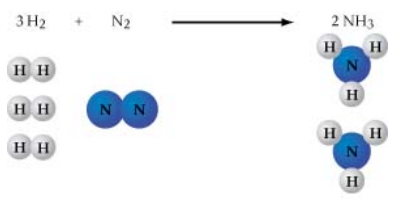# Problem: Now that we have put a coefficient of 3 in front of CaSO4, what coefficient should go in front of CaCl2 to balance calcium (Ca)?3 CaSO4 + AlCl3 → ?CaCl2 + Al2(SO4)3Express your answer numerically as an integer.According to the law of conservation of mass, matter cannot be created or destroyed. Therefore, a chemical equation must show the same number of each kind of atom in the reactants as it does in the products. As shown in the figure, the balanced equation 3 H2 + N2 → 2 NH3 has 6 atoms of hydrogen and 2 atoms of nitrogen on each side of the arrow. One is often presented with unbalanced chemical equations for which one must supply the coefficients.The following equation is not balanced:CaSO4 + AlCl3 → CaCl2 + Al2(SO4)3

###### FREE Expert Solution
90% (96 ratings)View Complete Written Solution
###### Problem Details

Now that we have put a coefficient of 3 in front of CaSO4, what coefficient should go in front of CaCl2 to balance calcium (Ca)?

3 CaSO4 + AlCl3 → ?CaCl2 + Al2(SO4)3

According to the law of conservation of mass, matter cannot be created or destroyed. Therefore, a chemical equation must show the same number of each kind of atom in the reactants as it does in the products. As shown in the figure, the balanced equation 3 H2 + N2 → 2 NH3 has 6 atoms of hydrogen and 2 atoms of nitrogen on each side of the arrow. One is often presented with unbalanced chemical equations for which one must supply the coefficients.The following equation is not balanced:

CaSO4 + AlCl3 → CaCl2 + Al2(SO4)3